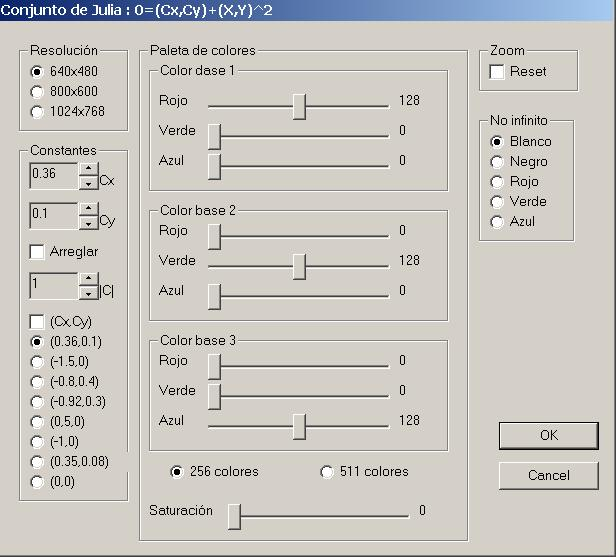Selected:

## Fractal geometry: Julia Sets

0,99

0,99

This software project is the resolution of the equation of set of Julia.

The download includes the application for Windows, the source code in C++ and Visual studio project

• Date: 2000
• Author: Raúl Bartolomé Castro

## Description

Fractal geometry is very present in nature, define the growth of many biological structures such as the lungs, neurons, the leaves of the trees. A fractal is an object attribute some of the following characteristics:

• It is too irregular to be described by conventional geometry.
• It has details on any scale of observation.
• Presents self similarity, e.g. its shape is similar from a distance or close up.
• Is defined by an algorithm (program) recursive.

I have made this introduction to discuss a program that I made in 2000 by solving the equation 0 = (Cx, Cy) + (x, y) ^ 2, which is a type of mathematical set of Julia. At that time I tried not to understand the profound impact of fractal geometry in everything that surrounds us, it was more like learning a programming language Visual C + +.

The following illustration can appreciate the application menu that appears when you right-click on the application.## Reviews

There are no reviews yet.

×

Cart